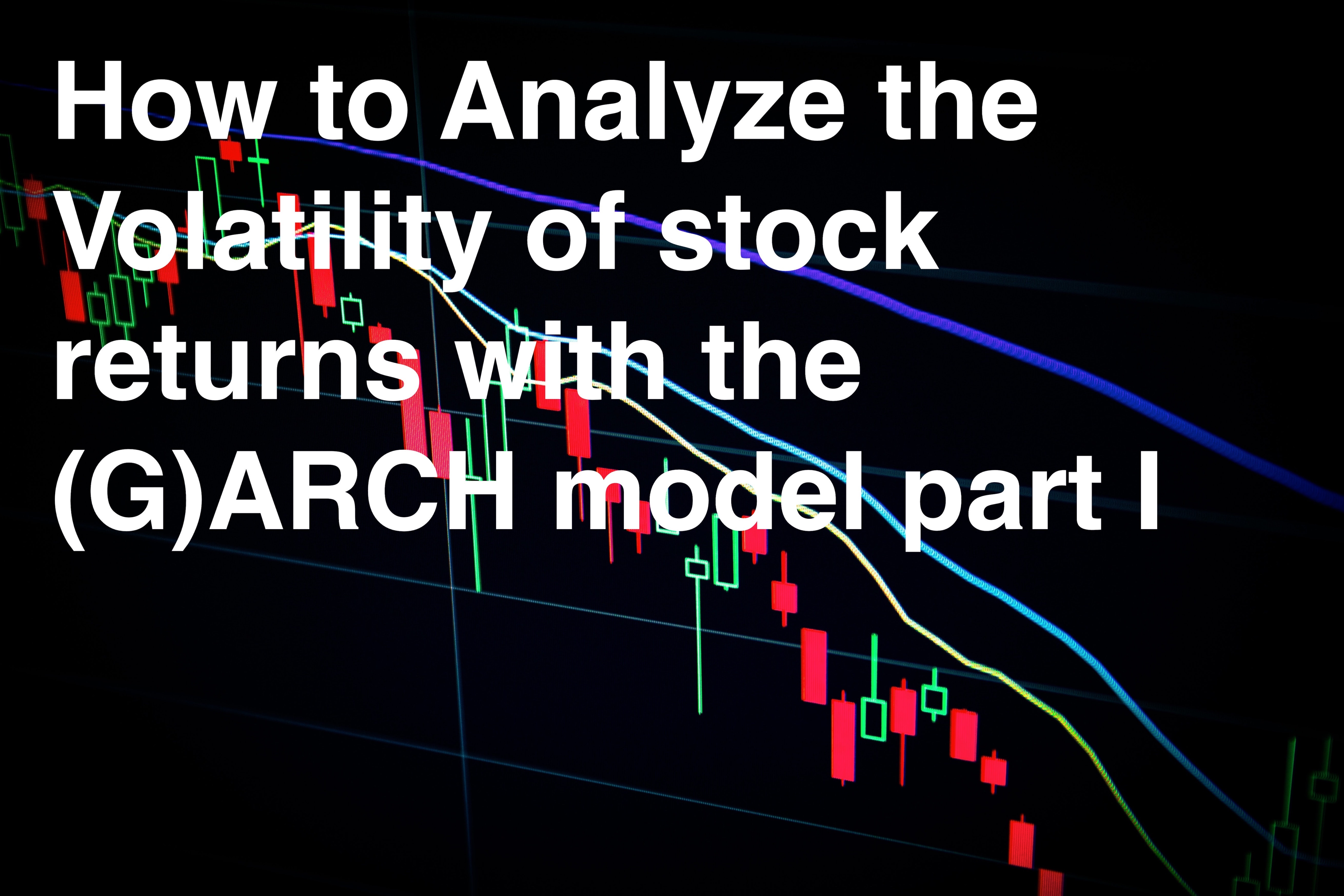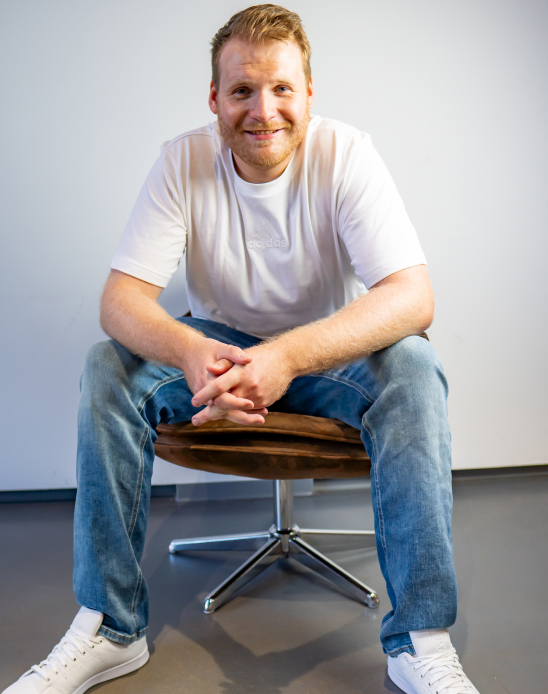10
min

# How to Analyze the Volatility of stock returns with the (G)ARCH model part I

Introduction of the AR/ARCH/GARCH-Model## 1. Motivation

Large fluctuations in financial markets have a strong impact on the returns of various asset classes. The random strong fluctuations are reflected in the volatility of returns. Since it is necessary to be able to optimally calculate or predict these fluctuations, the question of an adequate model arises. One of the relevant test models is the GARCH model developed by Tim Bollerslev, based on Robert F.Engle's ARCH model. These models allow modeling the volatility of time series. This work begins with an introduction to the data used and the asset classes used. This is followed by an explanation of the econometric methods. The focus here is on the AR model, ARCH model and theGARCH model. After this introduction I present an analysis of returns with discussion of skewness, kurtosis and autocorrelation. From this analysis I build fitted AR models and analyze the residuals with subsequent analysis ofARCH effects. After this discussion I introduce the standard GARCH model followed by an analysis of the residuals, ARCH effects and the presentation oft he conditional variance of the model. The conclusion is a final discussion on my part with reference to the most important results.

## 2. Data

Two time series data from different asset classes were selected. On the one hand the price development of gold as a commodity class and the price development of the Nasdaq100 Index. The data period runs from 01.12.2010 to01.12.2020. The number of observations is 2514. For the analysis of the data, the logarithmic returns were used to maintain stationarity. If weak stationarity can be implemented, the time series has the following properties(Stock/Watson 2019: 561f):

Since logarithmic returns are used throughout the analysis, the properties of weak stationarity can be assumed, at least in the analysis(Stock/Watson 2019: 561f).

## 3. Econometric methods

### 3.1 AR (Autoregressive Model)

This chapter explains the statistical methods that will later be used to analyze the data. First, the AR model will be explained. The p-th order autoregressive [AR(p)] model represents the conditional expectation of Y(t) as a linear function of p of its past values (Stock/Watson 2019: 567f):

The number of lags p is called the order or lag length of the autoregression (Stock/Watson2019: 567f).

### 3.2 ARCH(Autoregressive Conditional Heteroskedasticity Model)

ARCH stands for autoregressive conditional heteroskedasticity and is a model that estimates the evolution of variance over time. Its extension is the GARCH model. In the ARCH model, the error u(t) is modeled as normally distributed with zero mean and variance sigma^2(t), where sigma^2(t) depends on the past quadratic values of u(t) (Stock/Watson 2019: 669f). A special ARCH-model of height p (ARCH(p)) can be represented as follows (Stock/Watson 2019: 670):

If these coefficients are positive, the ARCH-model predicts that the current squared error will be large in the sense that its variance sigma^2(t) is large if the recent squared errors are large (Stock/Watson 2019: 670f). The ARCH-model can be applied to the error variance of any time series regression where the mean error equals zero, including higher-order ADL models (Stock/Watson 2019: 670f).

### 3.3 GARCH (Generalized Autoregressive Conditional Heteroskedasticity Model)

The GARCH model extends the ARCH model so that sigma^2(t) depends both on its own lags and on lags of the squared error (Stock/Watson 2019: 670f). The GARCH (p, q) model is:

The GARCH model can capture slowly changing variances with fewer parameters than the ARCH model (Stock/Watson2019: 670f). A large area of application of ARCH and GARCH models is the prediction of changes in the volatility of stock returns.

if you want to continue reading go to part II

Sources:

Stock, J.H.and Watson, M.W. (2019), Introduction to Econometrics, 4th Globaledition, Pearson Education Limited.

Image: from Maxim Hopman at Unsplash## Joshua Denefleh

CQO & Co-Founder

Strong background in financial markets, graduate from University Mannheim in B.Sc. Economics, KIT Karlsruhe University. Experience in quantitative methods, programming algo /w python